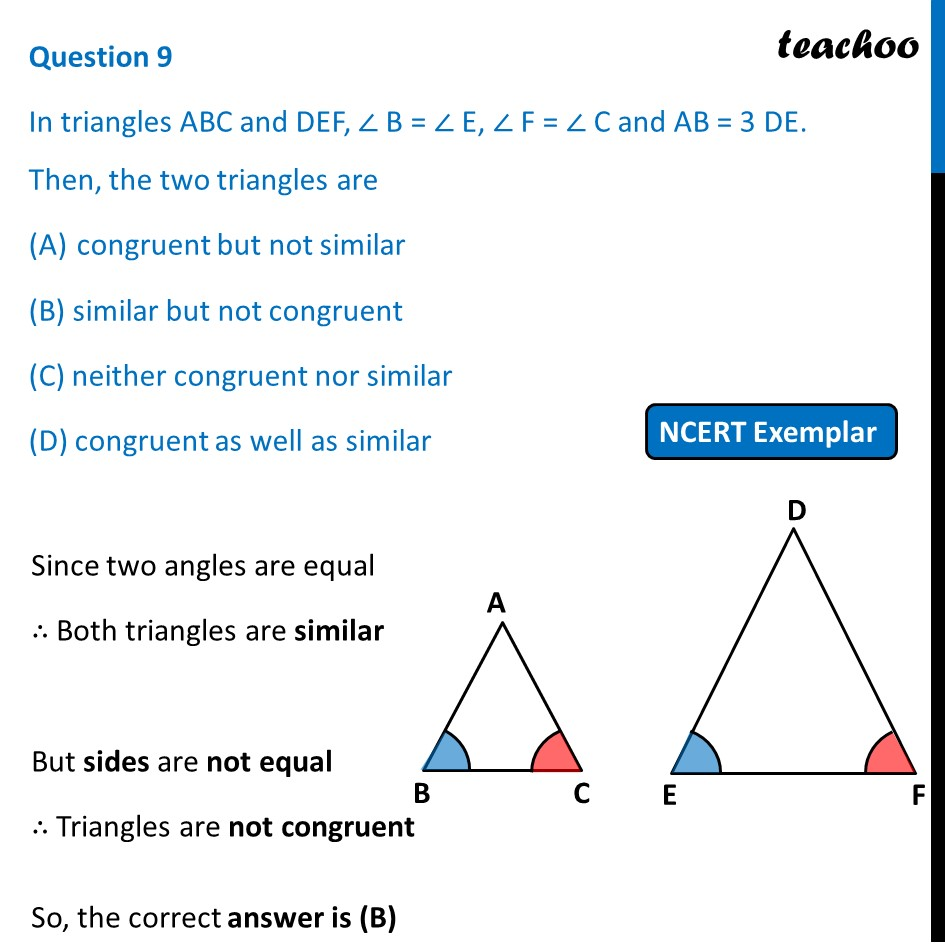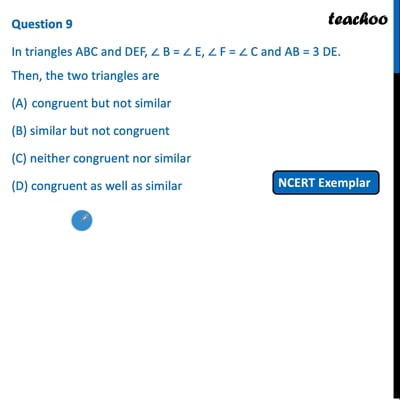NCERT Exemplar - MCQ

Chapter 6 Class 10 Triangles
Serial order wise

## (D) congruent as well as similarThis video is only available for Teachoo black users

Introducing your new favourite teacher - Teachoo Black, at only ₹83 per month

### Transcript

Question 9 In triangles ABC and DEF, ∠ B = ∠ E, ∠ F = ∠ C and AB = 3 DE. Then, the two triangles are congruent but not similar (B) similar but not congruent (C) neither congruent nor similar (D) congruent as well as similar Since two angles are equal ∴ Both triangles are similar But sides are not equal ∴ Triangles are not congruent So, the correct answer is (B)